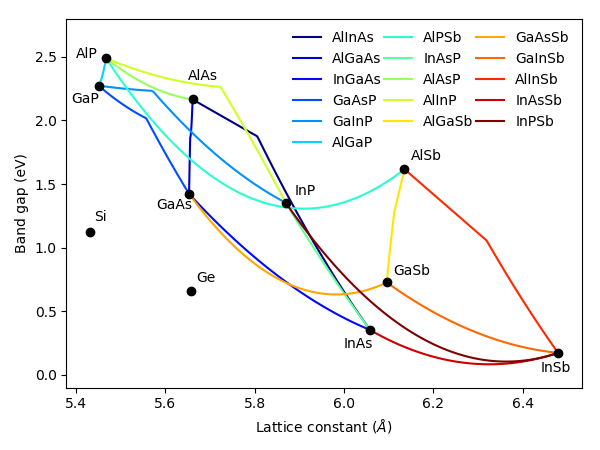# The Material System¶

The parameters database contains the basic properties of many semiconductor materials, including silicon, germanium and many III-V semiconductor binary and ternary alloys. Among other parameters, it includes the energy bandgap, the electron and hole effective masses, the lattice constants and the elastic constants.

The main sources of data are the article by I. Vurgaftman on Band parameters for III-V semiconductors () and the Handbook Series on Semiconductor Parameters by Levinshtein et al.(). The carrier mobility calculator is based on the empirical low-field mobility model by Sotoodeh et al. () and it is available only for some materials where the inputs for the model are available.

There are two methods for retrieving parameters from the database. The first one consists simply of getting the data using the `get_parameter` function with the required inputs. For example:

```get_parameter("GaAsP", "band_gap", P=0.45, T=300)
```

will return the bandgap of GaAsP for a phosphorus concentration of 45% at a temperature of 300 K, equal to 1.988 eV. This method only uses the existing data. Another method is to create a material object which will contain all the properties existing in the database for that material, as well as those included as input, which will override the value of the database parameter, if it exists. The following example creates a GaAs object and an AlGaAs object, using a custom electron effective mass in the latter:

```GaAs = material("GaAs")(T=300, Na=1e24)
AlGaAs = material("AlGaAs")(T=300, Al=0.3, Nd=1e23, eff_mass_electron=0.1)
```

Now, any parameter - including the custom ones - are attributes that can be easily accessed and used anywhere in the program. For example `GaAs.band_gap` is the GaAs bandgap and `AlGaAs.lattice_constant` is the AlGaAs lattice constant, both at the composition and temperature chosen when creating the objects.

The image below shows the well-known bandgap vs. lattice constant map of all semiconductor materials and alloys (only ternary compounds) currently implemented into Solcore. However, any other material can be used in all of the Solcore functions, as long as the necessary input parameters are provided. This can be done by overriding all the properties of an existing material during the creation as above, or adding it as an external material in the configuration files.## The mobility module¶

This module allows to calculate the carrier mobilities based on the material composition, temperature (T>100K) and impurity concentration. It is an implementation of the mobility model by Sotoodeh et al. (). The material class described above uses this module internally to get the mobililty of the materials it is implemented for.

The material parameters used in the model are included in the file mobility_parameters.json. At the moment, the supported materials are:

• Binaries: AlAs, GaAs, InAs, InP, GaP

• Ternaries: InAlAs, AlGaAs, InGaAs, GaInP, (GaAsP), (InAsP)

• Quaternaries: InGaAsP

The last two ternaries are simply calculated as a linear interpolation of the parameters of the corresponding binaries, so the transition from direct to indirect bandgap and other dependencies might not be very accurate. InGaAsP, in turn, is calculated only based on the InGaAs and GaInP data and it is mostly valid for compositions lattice matched to InP.